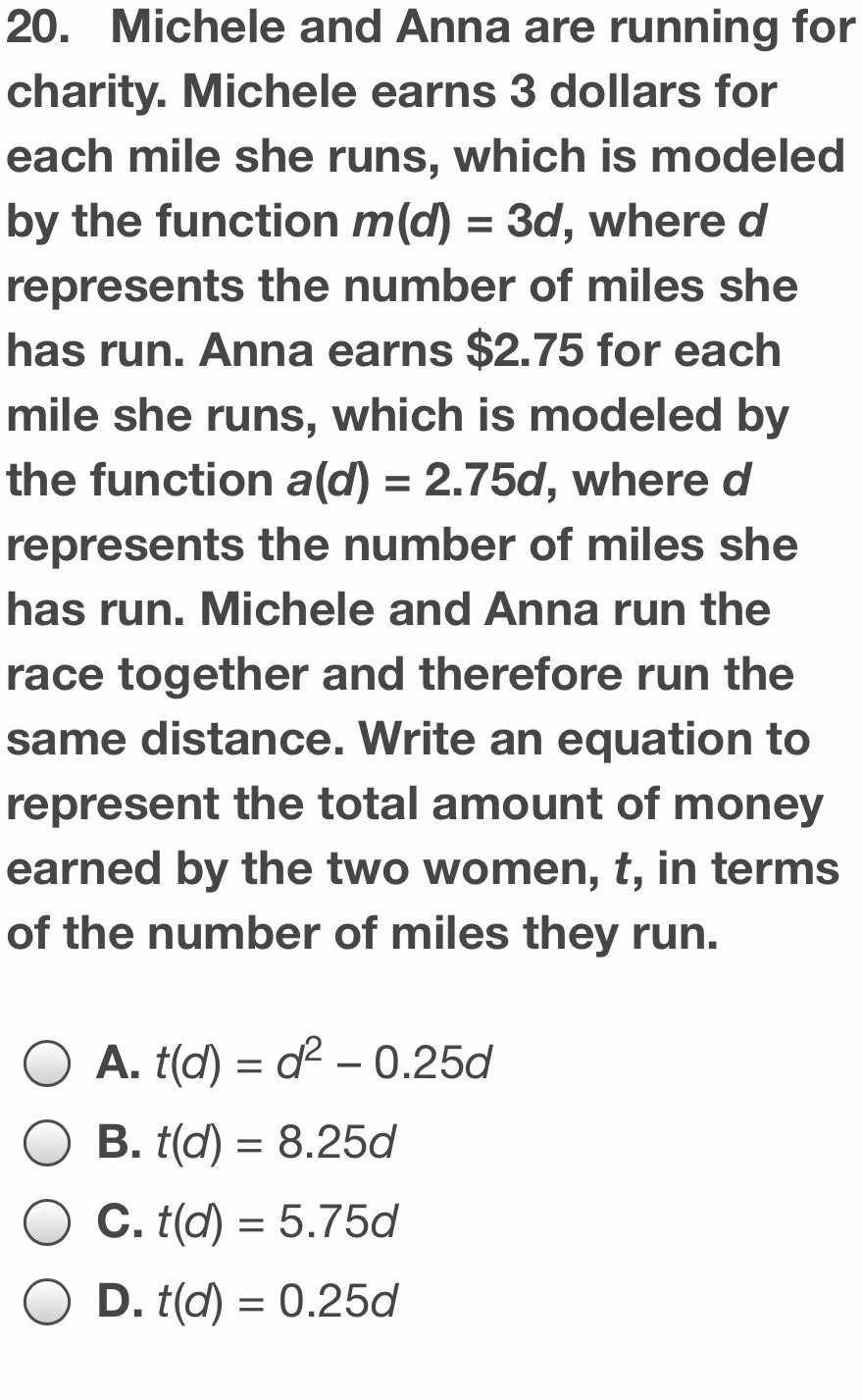### ¿Todavía tienes preguntas de matemáticas?

Pregunte a nuestros tutores expertos
Algebra
Pregunta20. Michele and Anna are running for charity. Michele earns $$3$$ dollars for each mile she runs, which is modeled by the function $$m ( d ) = 3 d ,$$ where $$d$$ represents the number of miles she has run. Anna earns $$\ 2.75$$ for each mile she runs, which is modeled by the function a(d) $$= 2.75 d ,$$ where $$d$$ represents the number of miles she has run. Michele and Anna run the race together and therefore run the same distance. Write an equation to represent the total amount of money earned by the two women, $$t$$ , in terms of the number of miles they run.

A. $$t ( d ) = d ^ { 2 } - 0.25 d$$

B. $$t ( d ) = 8.25 d$$

C. $$t ( d ) = 5.75 d$$

D. $$t ( d ) = 0.25 d$$